﻿ Is CMB just an Observational Effect of a Universe in Accelerated Expansion?

### Is CMB just an Observational Effect of a Universe in Accelerated Expansion?

Arne BergstromOPEN ACCESSPEER-REVIEWED

## Is CMB just an Observational Effect of a Universe in Accelerated Expansion?

Arne BergstromB&E Scientific Ltd, BN25 4PA, United Kingdom

### Abstract

Consider a universe in which an observer is surrounded by an infinite, roughly uniform distribution of luminous objects (stars or primeval stars), and assume further that each such object is opaque to those directly behind it. To the observer it would then seem as if he is surrounded by a distant, opaque wall emitting radiation. In addition, the requirement of Lorentz-covariant quantum transport has been shown to force the universe to be perceived to be in a state of apparent, exponentially accelerated expansion, producing redshifts in the spectra of the individual distant objects that together form the cosmic microwave background (CMB). The present article illustrates that under these two conditions, the observer would perceive the apparent distant luminous wall around him to radiate with an accurate blackbody spectrum, as observed. This suggests that the cosmic microwave background radiation might thus possibly be just an observational effect of this type (with traditional blackbody radiation as a limiting case), and hence may not necessarily have any connection to how the universe was created.

### At a glance: Figures

12
Prev Next

• Bergstrom, Arne. "Is CMB just an Observational Effect of a Universe in Accelerated Expansion?." International Journal of Physics 1.6 (2013): 133-137.
• Bergstrom, A. (2013). Is CMB just an Observational Effect of a Universe in Accelerated Expansion?. International Journal of Physics, 1(6), 133-137.
• Bergstrom, Arne. "Is CMB just an Observational Effect of a Universe in Accelerated Expansion?." International Journal of Physics 1, no. 6 (2013): 133-137.

 Import into BibTeX Import into EndNote Import into RefMan Import into RefWorks

### 1. Introduction

The study of the cosmic blackbody radiation as discussed in this article fits into a bigger picture as follows. The general time-dependent propagation of electromagnetic quanta through space is governed by a rigorous continuity equation (the Boltzmann transport equation). Requiring only that this equation should be equivalent to a Lorentz-covariant and dispersion-free wave equation  can be shown to lead to the following implications :

a. The universe is subjected to an apparent uniform expansion in agreement with Hubble’s law.

b. The quantum propagation from distant sources is subjected to an apparent quantum multiplication process, assumed to be the source of dark energy.

c. The uniform expansion in (a) is exponentially accelerating with time. This accelerated expansion and the dark energy created in (b) are two independent consequences of the Lorentz-covariant transport and are thus not dynamically connected to each other.

According to this approach, dark energy and the accelerated expansion would be independent effects of the requirements of a Lorentz-covariant quantum propagation, and are hence not dynamically connected: The accelerated expansion is thus not driven by any pressure from the dark energy, which thus eliminates the crucial problem of understanding the huge discrepancy by more than sixty orders of magnitude in the conventional picture . To the above three properties, the present article will here be shown to supplement the following property:

d. The exponentially accelerated expansion in (c) leads to a cosmic microwave background radiation with an exact blackbody spectrum (as observed ).

The present article discusses the observation that an infinite, roughly uniform distribution of extended, opaque objects of roughly the same size will be perceived from a point inside this distribution as being limited by an opaque wall at some finite distance away. This is for instance how the trees in a forest seemingly coalesce to an impenetrable wall around you at some distance away, or how you seem to be enclosed by a white sphere when flying in a cloud or being in a fog.

Similarly, this would also be how an assumed infinite, roughly uniform distribution of (primeval) stars would appear as seen from somewhere inside this distribution  thus possibly being the source of the apparent, roughly homogeneous cosmic microwave background we observe around us . In this picture, the cosmic microwave background is thus assumed to be caused by such an apparent wall of very old stars rather than by a big bang.

This article presents simulations of the energy spectrum from such a distribution of stars, assuming only (i) that a closer star completely obstructs the light from more distant ones in the same direction, and (ii) that the spectra of distant such stars appear redshifted as if subjected to an exponentially accelerated expansion  as derived in (c) above and as is also observed.

It will be shown below that under these two conditions, the energy spectrum from an infinite, roughly uniform distribution of such stars will be observed as an exact blackbody spectrum in agreement with astronomical observations. The cosmic microwave background radiation might thus possibly be just an observational effect of this type, and may hence not necessarily have any cosmological significance.

It should be remarked that the assumed obstruction by closer stars of the emission from more distant ones in the same direction is crucial for the formation of the blackbody spectrum discussed here. If closer stars were instead (unphysically) considered to be completely transparent to the emission from more distant ones, then the spectrum would, as illustrated in Figure 5 below, instead be a Gaussian distribution (as expected), not a blackbody spectrum.

Similarly, the assumed exponentially accelerated expansion is also crucial for the formation of the accurate blackbody spectra discussed here. Other forms of expansions will give distorted blackbody spectra, as will also be illustrated in Figure 5 below.

It should finally be remarked that what is considered here are deep-space distributions of stars such that the possibility of large contributions from individual, much closer stars or galaxies can be neglected.

### 2. Computer Simulation – Overview

As a consequence of the deep-space emission considered in this article as stated above, the width (and thus spectral form) of the individual spectra of the distant stars considered here can be neglected in comparison with their corresponding, much larger redshifts in the calculations below.

The spectra discussed in this article are calculated using Monte Carlo simulations as follows. Define P(x) as the angular distribution of radiation energy received by an observer at r = 0 from stars at different distances r as function of direction x in the sky, and assume closer stars (smaller r) to completely obstruct the emission from more distant ones in the same direction x as discussed above.

It is practical to arrange the directions x in the sky such that the radiation data are stored in successive locations in a one-dimensional table P[x], where x is an index describing angular elements that together cover all directions in the (small) part of the sky being considered.

Consider stars within a maximum distance R, and assume that a star at distance r R always subtends less than one location P[x] as seen from the observer at r = 0, and that it always obliterates anyone more distant star in the same P[x].

Now loop over L cases where angular positions x are sampled from a rectangular frequency function. Also sample distances r from a suitable radial frequency function (as will be discussed below), and calculate each corresponding energy contribution Q ∝ R2/r2 R/r, i e Q ∝ R3/r3, with closer radii r thus giving higher contributions, and the factor R/r being the redshift correction. (The exact positive power of R/r in Q turns out not to be critical in the simulations below, see comment below).

Then replace P[x] by Q if the new Q is greater than previous P[x], the new value thus corresponding to a smaller r and thus obliterating previous P[x] as discussed above.

Finally, loop over x and accumulate the energy spectrum S[P[x]] received by the observer, and compare with a Planck distribution.

Figure. 1a. Simulated received radiation energy P[x] accumulated (105 histories) from different directions x in a small region of the sky (with x = 0 … 10,000), and with closer sources assumed to be completely opaque to the radiation from more distant ones in the same direction. The corresponding spectrum is shown in Figure 1b to be essentially indistinguishable from an exact Planck spectrum. b. Energy spectrum S[ω] (suitably scaled, with ω = 10 … 100) accumulated (30105 histories) from the received radiation energy P[x] in Figure 1a. The sampled spectrum (black) is seen to be virtually indistinguishable from the Planck spectrum (red) also shown

Figure 1a shows the typical result for P[x] plotted as function of angular position x, in this case with x = 0 … 10,000 and L = 100,000.

Figure 1b shows the corresponding spectrum distribution S[ω], where 30 such samplings of P[x] are accumulated in a histogram over energy ω. Note that the simulated spectrum is virtually indistinguishable from the Planck spectrum also plotted in the figure.

### 3. Computer Simulation – Sampling Distance r

Above, the calculations of the radiation energy P[x] received by the observer were described. However, in this description the question of the sampling of the radial frequency function in order to get the path length to the next collision was explicitly left open. This question will now be addressed.

As discussed above, the universe is in this article considered to be in a state of exponentially accelerated expansion in accordance with implication (c) in the list described in the beginning. It should be emphasised again that this accelerated expansion, and its consequences as discussed in this article, have in this case nothing to do with properties derived from astronomical observations they are instead implications of very fundamental requirements on the propagation of the quanta involved .

Figure. 2a. Plot showing successive exponential expansions of type r(t) = exp(t - t0) -1 (green) for different (past) values of t0 ≥ 0, together with the trajectory of a light ray (red) through the present (defined as r = 0, t = 0). The choice of r = 0 and t = 0 as the present is arbitrary; it is a property of the exponential function that the curves would look the same if plotted for any other r and t chosen to define the present. (To the observer, both space and time appear subjected to exponential transformation ). b. Detail of Figure 2a around t = -1 and r = -1 showing how the set of exponential functions in Figure 2a seemingly form an essentially horizontal set of lines parallel to the t-axis, with decreasing separation for successively more distant r from the observer at r = 0 and piling up at r = -1. This property of exponential functions is important for the formation of a Planck spectrum as is illustrated in Figure 5 below

An exponential expansion of type exp(t - t0) is in this way deduced  from light emitted at previous times t0 (with t0 ≥ 0). Specifically, the times t0 are given by the intersections in Figure 2a when the exponential expansions in (1) below coincide with the particular light ray (with velocity c = 1) from the past in (2) that passes through the origin representing the present. This gives an observed density distribution of stars, which can be calculated from the following two simultaneous equations,(1)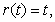(2)

where (1) describes the exponential expansions in the past (t0 > 0) and present (t0 = 0, with r(0) = 0), and (2) is a light-ray through the present.

Equations (1) and (2) above have the following solution expressed in the LambertW function  of emission time t0,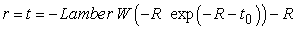(3)

Define z as the value of |r| above r = -R, where the exponential trajectories in Figures 2a and 2b pile up, i e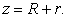(4)

Equation (3) then gives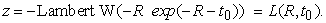(5)

The cumulative distribution function F(R, t0) = ∫z(t0 )dt0 corresponding to the frequency function defined by (5), and after normalisation, can be integrated to be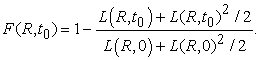(6)
Figure. 3. The cumulative distribution function F(R, t0) in (6) as function of t0 for values of R = 1, 2, 3, 4…, illustrating that for values of R greater than R ≈ 5, the function F(R, t0) is well approximated by the function F(t0) = 1 – exp (-t0 ) in (7)

The function (6) rises quickly from F = 0 at t0 = 0 to close to the asymptotic value F = 1 even for moderate t0. For R ≥ 5, the cumulative distribution function F(R, t0) in (6) is essentially independent of R, and is virtually indistinguishable (cf Figure 3) from the function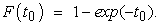(7)

Equation (7) can thus be used to simplify the sampling of values for t0 in the Monte Carlo simulations described above by sampling the inverse of (7) using random numbers from a rectangular probability function P (i e for which P = 1- P) as follows,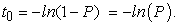(8)

Corresponding to (8), the radii r used in the simulations can thus be sampled from an expression of the following type, cf (4),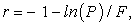(9)

where Φ is a positive scale factor determining how much the distribution is to be pushed towards the asymptotic value r = −1 in Figure 2a. From (8) we see that Φ corresponds to a scale factor in t0 and thus in t. The quantity Φ is here chosen in the range of, e g, 100 to 1000; note that then 1 + ln(P) /ΦP 1/Φ , which as remarked above explains why the exact power of R/r in Q and P is not critical, since it thus becomes emulated into Φ.

Figure. 4. Plot of the function F1 = -1 - ln(P)/1000 as in (9) [and for comparison F2 = -P1/1000 (coinciding)], illustrating that this function gives only a small variation in the value of r when P varies, which is the reason for the discussed piling-up at r = -1

### 4. Spectra from Different Emission Models

In order to give a perspective on the results presented in Figure 1b earlier, Figure 5 shows the energy spectra (normalised to same maximum) for three different assumptions on the emitted light from an ensemble of distant stars.

Case A shows a deep-space spectrum in the (unrealistic) case that closer stars were to let through the light from more distant ones in the same direction without any obstruction. This is thus in contrast to the corresponding spectrum in Case B (and Figure 1b discussed earlier), where light from more distant stars is assumed to be completely obstructed by closer stars in the same direction. In Case A, the simulations performed give a Gaussian spectral distribution  (normal distribution) of the following form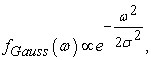(10)

where ω is a measure of the energy, and σ is the standard deviation.

The Gaussian distribution thus appearing in this case is to be expected, since according to the central limit theorem  the sum of a large number of random variables will under very general conditions approximate such a Gaussian distribution. Among these conditions is a requirement that the different contributions from the set of random observations all have the same (but not necessarily known) distribution.

Figure. 5. Sampled (108 histories) energy spectra S[ω] as in Figure 1b for A) a Gaussian distribution (galaxies transparent), B) a Planck distribution (galaxies opaque, exponentially accelerated expansion), and C) a Planck-type distribution with distorted tail (galaxies opaque, but no accelerated expansion)

This is thus a condition that is not fulfilled in the case of the assumed complete obstruction by closer stars of the emission from more distant ones in the same direction discussed in Case B. In Case B (and Case C below) this thus gives a distribution that is different from a Gaussian distribution as in Case A. In Case B this is, as discussed earlier, thus a Planck spectrum (blackbody spectrum) of the form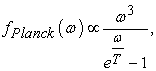(11)

describing the energy distribution of the emitted radiation at absolute temperature T.

The relation of Case B to standard blackbody radiation can be understood as follows. Consider a cavity in a furnace with high enough temperature for it to radiate with a peak wavelength corresponding to visible light. At this temperature, the cavity looks like a glowing surface with all details further inside the cavity obliterated. However, light of slightly shorter wavelengths may still penetrate a tiny, exponentially decaying distance from deeper inside the glowing cavity. These conditions correspond to the situation discussed above in Case B, and leading to a blackbody spectrum. Standard blackbody radiation thus corresponds to Case B in the limit when the quantity 1/Φ in equation (9) above is infinitesimally small.

Case C, finally, is again a case where closer stars are assumed to obstruct the light from more distant ones in the same direction as in Case B. In contrast to Cases A and B, however, in Case C there is assumed to be no exponentially accelerated expansion to press the star distribution towards more distant radii. A higher proportion of the radiation received by the observer atr = 0 in Case C has thus emanated from stars at closer distances than in Case B. This causes the tail end of the observed spectrum (corresponding to slightly higher received energies, i e from smaller |r|) in this case to be substantially higher than for the blackbody spectrum in Case B (and previous Figure 1b).

### 5. Concluding Remarks

The present analysis shows that a cosmic microwave background radiation would have a blackbody spectrum under the two conditions (i) that closer stars completely obstruct the light from more distant ones in the same direction, and (ii) that the universe is in a state of accelerated expansion (as theoretically required  and also observed [9, 10]). This means that a cosmic microwave background as the one observed may thus not necessarily be of cosmological origin, but might possibly be more an optical effect, as discussed in this paper.

The present analysis may also have some bearing on the so-called horizon problem (cf , where also some problems with the connection between inflation and CMB are discussed). The cosmic microwave background as described in this communication, as well as the apparent exponential expansion of the universe , are here considered to be effects related to the observer and thus be essentially centred around him. This may thus be part of several subjective requirements as discussed in the beginning of this article, and which an observer might intuitively impose in order to be able to make consistent observations of the world around him.

### References

  A. Bergstrom, “Relativistic invariance and the expansion of the universe”, Nuovo Cimento 27B, 145 (1975).In article  A. Bergstrom, “Lorentz-covariant quantum transport and the origin of dark energy”, Phys. Scr. 83, 055901 (2011).In article  J. Frieman, M. Turner, and D. Huterer, “Dark Energy and the Accelerating Universe”, Ann. Rev. Astron. Astrophys. 46 385 (2008).In article  D. L Fixsen, E. S. Gheng, J. M. Gales, J. C. Mather, R. A. Shafer, and E. L. Wright, “The cosmic microwave background spectrum from the full COBE FIRAS data set”, Astrophys. J. 473, 576 (1996).In article  P. S. Wesson, “Olbers's paradox and the spectral intensity of the extragalactic background light”, Astrophys. J. 367, 399 (1991).In article  R. M. Corless, G. H. Gonnet, D. E. G. Hare, D. J. Jeffrey, and D. E. Knuth, “On the Lambert W Function”, Adv. Comput. Math. 5, 329 (1996).In article  W. Feller, An Introduction to Probability Theory and Its Applications, Vol 1 Ch 7 (Wiley, 1970).In article  H. Fischer, A History of the Central Limit Theorem (Springer, 2011).In article  S. Perlmutter et al., “Measurements of Ω and Λ from 42 High-Redshift Supernovae”, Astrophys. J. 517, 565 (1999).In article  A G Riess et al., “Observational Evidence from Supernovae for an Accelerating Universe and a Cosmological Constant”, Astron. J. 116 1009 (1998).In article  G. D. Starkman and D. J. Schwarz, “Is the universe out of tune?”, Sci. Am. 293(2), 48 (2005).In article